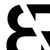# How to update existing Excel .xlsx file using Openpyxl?

3233
2
06-07-2021 07:25 PMby
New Contributor II

I am trying to make this code work, the problem is that in the excel file the print (...) data is not written, I have tried to use ws.appened (...) but without results.

``````import arcpy
from openpyxl import Workbook
wb = Workbook("C:/Users/Hp/Desktop/ejemplo/VINCULACION_S.xlsx")
ws =  wb.active
rows = arcpy.SearchCursor("C:/Users/Hp/Desktop/ejemplo/VH_Dissolve.shp",
fields="COLOR; INTERNO_DE; CLASE_DEMA; COUNT_AREA; SUM_AREA; SUM_LENGTH",
sort_fields="COLOR 222; INTERNO_DE A")
# COLOR, INTERNO_DE, CLASE_DEMA, COUNT_AREA, SUM_AREA y SUM_LENGTH.
for row in rows:
print("Color: {0}, Interno: {1}, Clase:{2}, ContarA: {3}, SumarA: {4}, SumarL: {5}".format(
row.getValue("COLOR"),
row.getValue("INTERNO_DE"),
row.getValue("CLASE_DEMA"),
row.getValue("COUNT_AREA"),
row.getValue("SUM_AREA"),
row.getValue("SUM_LENGTH")))
wb.save('VINCULACION_S.xlsx')``````

I have also tried to locate the results data in the excel file from cell B3: G3 onwards but I can't find it.

Tags (2)
1 Solution

Accepted Solutionsby
New Contributor II

I got this code as a solution to the post

``````import arcpy
import openpyxl as px

def main():
ws = wb['VINCULACION_SH_NUE']
in_features = r"C:\Users\Hp\Desktop\Ejemplo\VH_Dissolve.shp"

row_num = 3
with arcpy.da.SearchCursor(
in_features,
["COLOR", "INTERNO_DE", "CLASE_DEMA", "COUNT_AREA", "SUM_AREA", "SUM_LENGTH"],
) as cursor:
for row in cursor:
ws.cell(row=row_num, column=2).value = row
ws.cell(row=row_num, column=3).value = row
ws.cell(row=row_num, column=4).value = row
ws.cell(row=row_num, column=6).value = row
ws.cell(row=row_num, column=7).value = row
ws.cell(row=row_num, column=8).value = row
row_num += 1
wb.save(r"C:\Users\Hp\Desktop\Ejemplo\VINCULACION_S.xlsx")

if __name__ == "__main__":
main()``````
2 RepliesbyMVP Esteemed Contributor

Use data access cursors

SearchCursor—ArcGIS Pro | Documentation

And try your field list as a list, not a concatenated string

... sort of retired...by
New Contributor II

I got this code as a solution to the post

``````import arcpy
import openpyxl as px

def main():
ws = wb['VINCULACION_SH_NUE']
in_features = r"C:\Users\Hp\Desktop\Ejemplo\VH_Dissolve.shp"

row_num = 3
with arcpy.da.SearchCursor(
in_features,
["COLOR", "INTERNO_DE", "CLASE_DEMA", "COUNT_AREA", "SUM_AREA", "SUM_LENGTH"],
) as cursor:
for row in cursor:
ws.cell(row=row_num, column=2).value = row
ws.cell(row=row_num, column=3).value = row
ws.cell(row=row_num, column=4).value = row
ws.cell(row=row_num, column=6).value = row
ws.cell(row=row_num, column=7).value = row
ws.cell(row=row_num, column=8).value = row
row_num += 1
wb.save(r"C:\Users\Hp\Desktop\Ejemplo\VINCULACION_S.xlsx")

if __name__ == "__main__":
main()``````